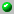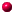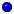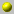Axel Kohnert

The homepage can be accessed here.

the following papers are available on our server= dvi format= ps format= tex format= pdf formatalgorithmen_fuer_plethysmenalgorithms_for_symmetric_functions_plethysmconstruction_of_linear_codes_with_prescribed_minimum_distancedominance_order_and_graphical_partitionsevaluation_of_modular_irreducible_matrix_representation_of_the_symmetric_groupsgraphdb_a_database_of_graphsmodular_irreducible_representations_of_the_symmetric_group_as_linear_codesmultiplication_of_a_schubert_polynomial_by_a_schur_polynomialschubert_polynomials_and_skew_schur_functionssymmetrica_an_object_oriented_computer_algebra_system_for_representations_combinatorics_and_applications_of_symmetrica_groupsthe_discovery_of_simple_7_designs_with_automorphism_group_pgammal_2_32the_discovery_of_simple_7_designs_with_automorphism_group_pgammal_2_32the_use_of_schubert_polynomials_in_symcharusing_schubert_toolkit_to_compute_with_polynomials_in_several_variablesweintrauben_polynome_tableaux

Go back to the directory of pre and reprints.
Or search the database by keywords
Or look for the newest entires in the database